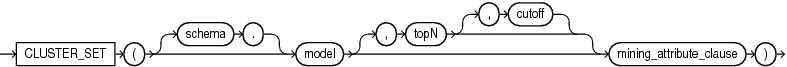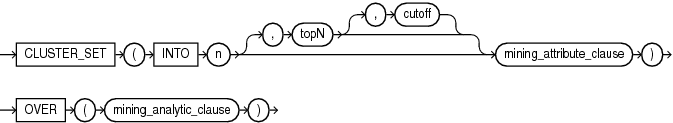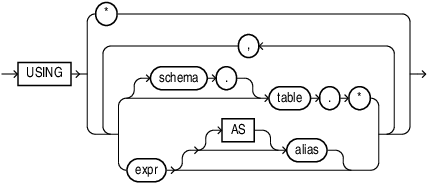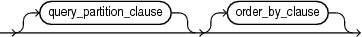## CLUSTER_SET

Syntax

cluster_set::=Description of the illustration cluster_set.eps

Analytic Syntax

cluster_set_analytic::=Description of the illustration cluster_set_analytic.eps

mining_attribute_clause::=Description of the illustration mining_attribute_clause.eps

mining_analytic_clause::=Description of the illustration mining_analytic_clause.eps

Analytic Functions for information on the syntax, semantics, and restrictions of `mining_analytic_clause`

Purpose

`CLUSTER_SET` returns a set of cluster ID and probability pairs for each row in the selection. The return value is a varray of objects with field names `CLUSTER_ID` and `PROBABILITY`. The cluster identifier is an Oracle `NUMBER`; the probability is `BINARY_DOUBLE`.

topN and cutoff

You can specify `topN` and `cutoff` to limit the number of clusters returned by the function. By default, both `topN` and `cutoff` are null and all clusters are returned.

• `topN` is the `N` most probable clusters. If multiple clusters share the `N`th probability, then the function chooses one of them.

• `cutoff` is a probability threshold. Only clusters with probability greater than or equal to `cutoff` are returned. To filter by `cutoff` only, specify `NULL` for `topN`.

To return up to the `N` most probable clusters that are greater than or equal to `cutoff`, specify both `topN` and `cutoff`.

Syntax Choice

`CLUSTER_SET` can score the data in one of two ways: It can apply a mining model object to the data, or it can dynamically mine the data by executing an analytic clause that builds and applies one or more transient mining models. Choose Syntax or Analytic Syntax:

• Syntax — Use the first syntax to score the data with a pre-defined model. Supply the name of a clustering model.

• Analytic Syntax — Use the analytic syntax to score the data without a pre-defined model. Include `INTO` `n`, where `n` is the number of clusters to compute, and `mining_analytic_clause`, which specifies if the data should be partitioned for multiple model builds. The `mining_analytic_clause` supports a `query_partition_clause` and an `order_by_clause`. (See analytic_clause::=.)

The syntax of the `CLUSTER_SET` function can use an optional `GROUPING` hint when scoring a partitioned model. See GROUPING Hint.

mining_attribute_clause

`mining_attribute_clause` identifies the column attributes to use as predictors for scoring. When the function is invoked with the analytic syntax, these predictors are also used for building the transient models. The `mining_attribute_clause` behaves as described for the `PREDICTION` function. (See mining_attribute_clause::=.)

Note:

The following example is excerpted from the Data Mining sample programs. For more information about the sample programs, see Appendix A in Oracle Data Mining User’s Guide.

Example

This example lists the attributes that have the greatest impact (more that 20% probability) on cluster assignment for customer ID 100955. The query invokes the `CLUSTER_DETAILS` and `CLUSTER_SET` functions, which apply the clustering model `em_sh_clus_sample`.

```SELECT S.cluster_id, probability prob,
CLUSTER_DETAILS(em_sh_clus_sample, S.cluster_id, 5 USING T.*) det
FROM
(SELECT v.*, CLUSTER_SET(em_sh_clus_sample, NULL, 0.2 USING *) pset
FROM mining_data_apply_v v
WHERE cust_id = 100955) T,
TABLE(T.pset) S
ORDER BY 2 DESC;

CLUSTER_ID  PROB DET
---------- ----- ------------------------------------------------------------------------------
14 .6761 <Details algorithm="Expectation Maximization" cluster="14">
<Attribute name="AGE" actualValue="51" weight=".676" rank="1"/>
<Attribute name="HOME_THEATER_PACKAGE" actualValue="1" weight=".557" rank="2"/>
<Attribute name="FLAT_PANEL_MONITOR" actualValue="0" weight=".412" rank="3"/>
<Attribute name="Y_BOX_GAMES" actualValue="0" weight=".171" rank="4"/>
<Attribute name="BOOKKEEPING_APPLICATION" actualValue="1" weight="-.003"rank="5"/>
</Details>

3 .3227 <Details algorithm="Expectation Maximization" cluster="3">
<Attribute name="YRS_RESIDENCE" actualValue="3" weight=".323" rank="1"/>
<Attribute name="BULK_PACK_DISKETTES" actualValue="1" weight=".265" rank="2"/>